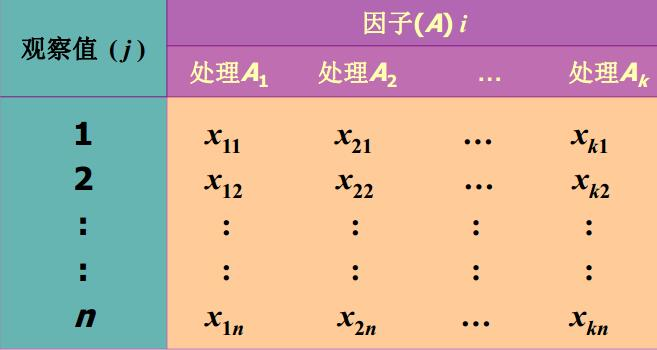# 第 8 章 ANOVA

## 8.1 方差分析的引论

• 一个或多个分类型自变量：两个或多个 (k 个) 处理或分类
• 一个数值型因变量

• 通过分析数据的误差判断各总体均值是否相等

• 单因子方差分析：涉及一个分类型自变量
• 双因子方差分析：涉及两个分类型自变量

（1）假设检验：一次只能研究两个样本

• 需要比较的次数随因子的数量增多而增多；
• 第一类错误发生的可能性增大。

（2）方差分析：同时分析多个样本

• 提高检验效率；
• 将所有信息结合在一起， 增加了分析的可靠性。

### 8.1.1 方差分析的部分概念

• 因子或因素 (factor)——所要检验的对象，要分析行业对投诉次数是否有影响， 行业是要检验的因子或因素。
• 水平或处理(treatment):因子的不同表现,零售业、 旅游业、 航空公司、 家电制造业就是因子的处理。
• 观察值：在每个因子处理下得到的样本数据，每个行业被投诉的次数就是观察值。
• 试验：涉及一个因子多水平， 可称为单因子多处理的试验。
• 总体：因子的每一个处理看作是一个总体。
• 样本数据：观察值可以看作是从着多个总体中抽取的样本数据

### 8.1.2 方差分析的基本思想和原理

• 随机误差——因子的同一处理(总体)下， 样本各观察值之间的差异，这种差异可以看成是随机因素的影响， 称为随机误差。
• 系统误差——因子的不同处理(不同总体)下，各观察值之间的差异，这种差异可能是由于抽样的随机性所造成的，也可能是由于行业本身所造成的，后者所形成的误差是由系统性因素造成的，称为系统误差。

• 组内平方和(within groups)——因子的同一处理(同一个总体)下样本数据的平方和。组内平方和只包含随机误差。
• 组间平方和(between groups)——因子的不同处理(不同总体)下各样本之间的平方和。组间平方和既包括随机误差， 也包括系统误差。

• 若原假设不成立， 组间平方和平均后的数值就会大于组内平方和平均后的数值， 它们之间的比值就会大于1。
• 当这个比值大到某种程度时， 就可以说不同处理之间存在着显著差异， 也就是自变量对因变量有影响。

### 8.1.3 方差分析的基本假定

（1）每个总体都应服从正态分布：对于因子的每一个处理， 其观察值是来自服从正态分布总体的简单随机样本。

（2）各个总体的方差必须相同：各组观察数据是从具有相同方差的总体中抽取的。

（3）观察值是独立的。

（4）在上述假定条件下， 判断行业对投诉次数是否有显著影响， 实际上也就是检验具有同方差的四个正态总体的均值是否相等。

（5）如果四个总体的均值相等， 可以期望四个样本的均值也会很接近：四个样本的均值越接近，推断四个总体均值相等的证据也就越充分；样本均值越不同， 推断总体均值不同的证据就越充分。

• n个水平被投诉次数的均值都相等；
• 意味着每个样本都来自均值为$$\mu$$、方差为$$\sigma^2$$的同一正态总体。

• 至少有一个总体的均值是不同的；
• 样本分别来自均值不同的多个个正态总体。

## 8.2 单因子方差分析（One-way ANOVA)• 提出假设；
• 构造检验统计量；
• 统计决策。

$$H_0:\mu_1=\mu_2=\mu_3=\cdots=\mu_n$$（自变量对因变量没有显著影响）。

$$H_1:\mu_1,\mu_2,\mu_3,\cdots,\mu_n$$不全相等（自变量对因变量有显著影响）。

（1）处理的均值

$\bar x_i=\frac{\sum_{j=1}^nx_{ij}}{n_i} (i=1,2,\cdots,k)$

（2）全部观察值的总均值

$\bar x'=\frac{\sum_{i=1}^k\sum_{j=1}^nx_{ij}}{n}=\frac{\sum_{i=1}^kn_i\bar x_i}{n}$

（3）平方和

（4）均方——构建检验统计量

$MSTR=\frac{SSTR}{k-1}$

$MSE=\frac{SSE}{n-k}$

$F=\frac{MSTR}{MSE}\sim(k-1,n-k)$

• 根据给定的显著性水平$$\alpha$$， 在F分布表中查找与第一自由度$$df_1=k-1$$、 第二自由度$df_2=n-k$ 相应的临界值$$F_\alpha$$
• 若F>$$F_\alpha$$，则拒绝原假设$$H_0$$，表明均值之间的差异是显著的，所检验的因子对观察值有显著影响。
• 若F<$$F_\alpha$$，则不能拒绝原假设$$H_0$$， 无证据支持表明所检验的因子对观察值有显著影响。

（1）提出假设；

（2）构造检验统计量（均值：全部观察值的总均值、处理的均值；平方和：总平方和SST，处理平方和SSTR，误差平方和SSE；均方：处理均方MSTR，误差均方MSE；均方比：MSTR/MSE~F分布）；

（3） 统计决策。

a.aov<-aov(value~factor,data=a)
summary(a.aov)

• 当处理平方和比误差平方和(SSE)大， 而且大到一定程度时， 就意味着两个变量之间的关系显著， 大得越多， 表明它们之间的关系就越强。 反之， 就意味着两个变量之间的关系不显著， 小得越多， 表明它们之间的关系就越弱。

## 8.3 方差分析中的多重比较

• 可采用Fisher提出的最小显著差异方法， 简写为LSD-least significant difference。LSD方法是对检验两个总体均值是否相等的t检验方法的总体方差估计加以修正（ 用MSE来代替） 而得到的。

（1）提出假设：$$H_0: \mu_i=\mu_j )$$(第i个总体的均值等于第j个总体的均值，$$H_1:\mu_i\neq\mu_j$$(第i个总体的均值不等于第j个总体的均值)；

（2）计算检验的统计量: $$\bar x_i-\bar x_j$$

（3）计算LSD,t分布的自由度为n-k（MSE的自由度为n-k）。$$LSD=t_{\alpha/2}\sqrt{MSE(\frac{1}{n_i}+\frac{1}{n_j})}$$

（4）决策：若$$\vert {\bar x_i-\bar x_j} \vert > LSD$$，拒绝$$H_0$$；若$$\vert {\bar x_i-\bar x_j} \vert < LSD$$，不拒绝$$H_0$$

## 8.4 双因子方差分析（Two-way ANOVA）

### 8.4.1 双因子方差分析的基本假定

（1） 每个总体都服从正态分布（对于因素的每一个水平， 其观察值是来自正态分布总体的简单随机样本）。

（2） 各个总体的方差必须相同（对于各组观察数据， 是从具有相同方差的总体中抽取的）。

（3） 观察值是独立的。

### 8.4.2 无交互作用双因子方差分析

• 设因子A有a个水平$$A_1,A_2,\cdots,A_a$$，因子B有b个水平$$B_1,B_2,\cdots,B_b$$，每组因子组合进行1次试验，其结果为$$x_{ij},x_{ij}\sim N(\mu_{ij},\sigma^2)$$,现在要研究它们对因变量X的影响。

（1）无交互作用双因子方差分析：模型

$X_{ij}=\mu+\alpha_i+\beta_j+\varepsilon_{ij}$

（2）无交互作用双因子方差分析：假设

（3）计算步骤（公式大全） > * 均方：

$$\bar x_{i.}$$是A因素的第i个水平下各观察值的平均值

$\bar x_{i.}=\frac{\sum_{j=1}^bx_{ij}}{b}(i=1,2,\cdots,a)$

$$\bar x_{.j}$$是B因素的第j个水平下各观察值的平均值

$\bar x_{.j}=\frac{\sum_{i=1}^ax_{ij}}{a}(i=1,2,\cdots,b)$

$$\bar x'$$是B因素的第j个水平下各观察值的平均值

$\bar x'=\frac{\sum_{i=1}^a\sum_{j=1}^bx_{ij}}{ab}$

• 平方和：

$SST=\sum_{i=1}^a\sum_{j=1}^b(x_{ij}-\bar x')^2$

$SSA=b\sum_{i=1}^a(x_{i.}-\bar x')^2$

$SSB=a\sum_{j=1}^b(x_{.j}-\bar x')^2$

$SSE=\sum_{i=1}^a\sum_{j=1}^b(x_{ij}-x_{i.}-x_{.j}+\bar x')^2$

$SST=SSA+SSB+SSE$

• 计算均方（MS）构造检验统计量：误差平方和除以相应的自由度

A因素的均方，记为MSA，计算公式为：

$MSA=\frac{SSA}{a-1}$

B因素的均方，记为MSB，计算公式为：

$MSB=\frac{SSB}{b-1}$

$MSE=\frac{SSE}{(a-1)(b-1)}$

• 计算检验统计量(F)

$F_A=\frac{MSA}{MSE}\sim F(a-1,(a-1)(b-1))$

$F_B=\frac{MSB}{MSE}\sim F(b-1,(a-1)(b-1))$

• 统计决策

### 8.4.3 有交互作用双因子方差分析

（1）有交互作用双因子方差分析：模型

$X_{ijk}=\mu+\alpha_i+\beta_j+(\alpha\beta)_{ij}+\varepsilon_{ijk}$

（2）交互作用双因子方差分析：假设

$SST=\sum_{i=1}^a\sum_{j=1}^b\sum_{k=1}^r(x_{ij}-\bar x')^2$

$SSA=br\sum_{i=1}^a(x_{i.}-\bar x')^2$

$SSB=ar\sum_{j=1}^b(x_{.j}-\bar x')^2$

$SSAB=r\sum_{i=1}^a\sum_{j=1}^b(\bar x_{ij}-\bar x_{i.}-\bar x_{.j}+\bar x')^2$

$SSE=\sum_{i=1}^a\sum_{j=1}^b\sum_{k=1}^r(x_{ijk}-\bar x_{ij})^2$

$SST=SSA+SSB+SSAB+SSE$

$F_A=\frac{MSA}{MSE}\sim F(a-1,ab(r-1))$

$F_B=\frac{MSB}{MSE}\sim F(b-1,ab(r-1))$

$F_{AB}=\frac{MSAB}{MSE}\sim F((a-1)(b-1),ab(r-1))$

$F_A> F_\alpha(a-1,ab(r-1))$

$F_B> F_\alpha(b-1,ab(r-1))$

$F_{AB}> F_\alpha((a-1)(b-1),ab(r-1))$

## 8.5 实验设计初步

（1） 因子数量（单因子方差分析，双因子方差分析……）；

（2） 因子处理的数量;

（3） 实验设计类型。

（1）完全因素位级组合（Full factorial design）

• 如果有k个因子，对于k个因子的第i个水平来说，会有$$n_i$$个水平的观测值：$$n=\prod_{i=1}^k n_i$$
• 必须实验每个可能的因子水平的组合。
• 必须捕获有关交互的全部信息。
• 大量的工作。

• 完全随机化设计(completely randomized design)——“处理” 被随机地指派给试验单元的一种设计，“处理” 是指可控制的因子的各个水平 “试验单元(experiment unit)”是接受“处理”的对象或实体。
• 随机化区组设计(randomized block design)——先按一定规则将试验单元划分为若干同质组， 称为“ 区组(block)”，再将各种处理随机地指派给各个区组,分组后再将每个品种（ 处理） 随机地指派给每一 个区组的设计就是随机化区组设计。如果可能， 我们应选择随机化区组设计

（2）部分因素位级组合（Fractional factorial design）

• 仅测量部分因子水平的组合的结果。
• 必须认真设计来捕获所有可能的交互作用。
• 相比而言，工作量降低了，不确定性增大了。
• 在知道一些因子不存在交互作用的前提下特别有效。